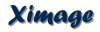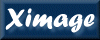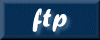## Tcl Scripting

Tcl is a scripting language which provides new capabilities to the ximage command line beyond the program-specific commands, allowing for example if-branching and looping which was previously unavailable to ximage scripts.

This page is a primer for Tcl scripting in ximage and contains only basic Tcl commands. For a full reference of all Tcl commands, see the Tcl manual (Use command "puts \$tcl_patchLevel" in ximage to determine version).

## Simple Syntax

Below is a simple example which illustrates the most basic functionality of the Tcl command line.

Assign 42 to variable x with the set command. In interactive mode (i.e. not running a script), the result of a command is always printed, 42 in this case.

```[XIMAGE> set x 42
42
```

The expr command must be used to evaluate math expressions, otherwise it will be interpretted as a string.

```[XIMAGE> expr 6*9
54
[XIMAGE> set y 6*9
6*9
```

Anything in square brackets [] is evaluated as a command and is substituted with its result.

```[XIMAGE> set y [expr 6*9]
54
```

The puts command prints to the screen. Anything of the form \$variable will be substituted with the variable value.

```[XIMAGE> puts "Value of x: \$x \nValue of y: \$y"
Value of x: 42
Value of y: 54
[XIMAGE> puts "Difference (y-x): [expr \$y - \$x]"
Difference (y-x): 12
```

If, however, a string must be quoted such that no substitutions occur, use curly-braces {}.

```[XIMAGE> puts {To access the value of x, use \$x}
To access the value of x, use \$x
```

## Script Execution

Below is an example which illustrates branching and looping syntax which may be useful in the context of a script file.

```#
#   Test script
#
set x 42
set y [expr 6*9]

!   if statement

if { \$x == \$y }  {
puts "It's true"
} else {
puts "It's false"
}

!   for statement
#   Note: "incr i" is a shortcut for "set i [expr \$i+1]"

for { set i 1 } { \$i <= 2 } { incr i } {
puts "Count \$i"
}

!   while statement

set i 0
while { \$i != 2 } {
incr i
}
puts "Final value: \$i"
```

Such a file containing ximage commands may be executed by preceding the script file name with @, which will echo the commands as they are executed. Note: # and ! both indicate a comment, however, # comments are silent, while ! comments are echoed. For example,

```[XIMAGE> @test.xco
![XIMAGE> set x 42
![XIMAGE> expr 6*9
![XIMAGE> set y [expr 6*9]
![XIMAGE> !   if statement
![XIMAGE> if { \$x == \$y }  {
![XIMAGE>  puts "It's false"
It's false
![XIMAGE> !   for statement
![XIMAGE> for { set i 1 } { \$i <= 2 } { incr i } {
![XIMAGE>  set i 1
![XIMAGE>  puts "Count \$i"
Count 1
![XIMAGE>  incr i
![XIMAGE>  puts "Count \$i"
Count 2
![XIMAGE>  incr i
![XIMAGE> !   while statement
![XIMAGE> set i 0
![XIMAGE> while { \$i != 2 } {
![XIMAGE>  incr i
![XIMAGE>  incr i
![XIMAGE> puts "Final value: \$i"
Final value: 2
```

The Tcl source command, however, runs the script commands without echoing them to the screen.

```[XIMAGE> source test.xco
It's false
Count 1
Count 2
Final value: 2
```

In particular, source is useful if you are defining new commands with the proc command. In the following example, after the proc is defined, the command qdisp image.fits will read and display image.fits, annotating it with a grid and scale.

```proc qdisp {file} {
disp
scale
grid
}
```

For further details on creating a new ximage command, including the capability to use "command qualifier=value" syntax, see Implementing New Commands

## Special Script Commands

txwrite message chat_level

The txwrite command prints the message only when the defined chat_level is less than or equal to the current chat level defined with the ximage chat command. By default the terminal and log chat levels are set to 10.

The txread command queries the user for a value giving the prompt as the question, returning the user's answer. Common usage: set answer [txread "question> "]

listclean \$var

The listclean command provides a simple way to support the standard Tcl list syntax as well as a commonly used comma-delimited format in ximage. If the string has spaces, it will be interpretted as a standard Tcl list, otherwise the results will be split by the ',' character. Common usage: set x [listclean \$x]

There are a number of standard ximage commands which are most useful in a scripting context: coord, draw, select, prarray and rdarray. See the ximage manual or use the help command at the ximage prompt for documentation.

## Global Tcl Variables

During an ximage session, there are global variables that are updated, reflecting information about current settings as well as global variables that may be redefined to change the way ximage behaves.

VariablePermissionsDescription
tchat/lchat Read-only terminal chat/log chat (set with chat command)
curmap/dismap Read-only current map/display map (set with map command)
scriptchan Read-only output channel set by script command
maxmaps Read-only maximum number of maps (9)
NULL Read-only value that represents NULL in image maps
ASTBAD Read-only value that represents an invalid coordinate (output by coord command)
savmode R/W save/restore on(1) or off(0)
savmap R/W saved mapid (MAP9 by default)
xtkcols R/W /xtk device colors
xtkwid R/W /xtk device width
xtkhgt R/W /xtk device height
grid_astoptions R/W Sets options to apply to grid command using AST syntax (see manual) (e.g. set grid_astoptions="Labelling=interior,Color(NumLab)=2"). See grid command documentation.
exit_on_startfailR/WIf set, ximage will exit with that value as its error code when a startup script fails
remap_method R/W Sets default remap method. See remap/method command docs
read_image_limitsizeR/W Sets limit of default image size. Set to 0 for no limit. See read_image/size command docs
ximage_history_fileR/W Sets the path to the ximage history file. Set to "" to write no history. Must be set in ~/.ximagerc

As of ximage 4.4, values saved between ximage sessions are no longer stored in the ~/defaults.def file, but rather in a standard parameter file called ximage.par. This parameter file may be manipulated or inspected using the standard pset and plist tools (e.g. plist ximage). The values in the parameter file are read into the global array default. This array may be set directly or through associated ximage commands. Upon exiting ximage, the values in the default array are stored in the parameter file.

VariableAssociated CommandDescription
default(device)cpdPlot device
default(equinox)ceyDefault equinox
default(numlevs)levels/num=valueNumber of image levels
default(svra)
default(svdec)
default(svequinox)
ra_dec_to_pixelSaved position

## Result-returning Commands

The internal header associated with each map and the mission database contain key values which may be useful when scripting. If the key qualifier is specified, and the value qualifier is not, the chheader and chmdb commands return the value of the key as the result of the command. For example,

```[XIMAGE> set telescop [chh map=cur key=telescop]
ROSAT
[XIMAGE> set ecol [chmdb tel=rosat ins=pspc key=ecol]
PI
```
Other commands that set a Tcl result:

 equinox Returns the current equinox isastbad [value] Returns whether (0/1) the supplied value is ASTBAD isnull [value] Returns whether (0/1) the supplied value is NULL map isequal [mapid] [mapid] Returns whether (0/1) mapids correspond to the same map map gettmp Returns a mapid representing a temporary map select Returns the selected detector coordinates in the form of a Tcl list

## Command Variables

Certain ximage commands create arrays with the same name as the command executed which contain values from the command, such as the results of calculations performed.

In Tcl, an array is indexed by strings and its value is substituted when preceded by a \$, for example: puts "Counts: \$counts(value)". A useful command for viewing arrays is array. For example, array names counts will list all the element names in the counts array, and array get counts lists all the element names in the counts array immediately followed by its value. Other ways of viewing the contents of an array include the Tcl command, parray (e.g. parray counts), and the Ximage-specific command, prarray (e.g. prarray counts).

Listed below are the commands that create an array and the contents of the array. The array's scope is either global, which means only one copy of the array exists for all contexts, or local, which means the array is only available in the current context.

BACKGROUND command (global)

```background(mapid)     = Map used in background calculation
background(imgpix)    = Background in counts/image-pixel
background(detpix)    = Background in counts/det-pixel
background(arcmin)    = Background in counts/sq-arcmin
background(detpixsec) = Background in counts/det-pixel/sec
background(arcminsec) = Background in counts/sq-arcmin/sec
```

CCT command (local)

```cct(current)    = Current color table
cct(bright)     = Current brightness
cct(contrast)   = Current contrast
cct(default)    = Default color table
cct(defbri)     = Default brightness
cct(defcon)     = Default contrast
```

CENTROID command (local)

```centroid(ra)       = Right ascension of centroid
centroid(dec)      = Declination of centroid
centroid(equinox)  = Equinox of RA/Dec
centroid(lii)      = Galactic longitude of centroid
centroid(bii)      = Galactic latitude of centroid
centroid(xpix)     = Detector x coordinate of centroid
centroid(ypix)     = Detector y coordinate of centroid
centroid(ximg)     = Image x coordinate of centroid
centroid(yimg)     = Image y coordinate of centroid
```

COORD command (local)

```coord(system)   = Name of coordinate system for x/ysky
coord(xsky)     = Sky x position
coord(ysky)     = Sky y position
coord(xsfmt)    = Sky x position in printable format
coord(ysfmt)    = Sky y position in printable format
coord(ra)       = Right ascension of position
coord(dec)      = Declination of position
coord(equinox)  = Equinox of RA/Dec
coord(lii)      = Galactic longitude of position
coord(bii)      = Galactic latitude of position
coord(xpix)     = Detector x coordinate of position
coord(ypix)     = Detector y coordinate of position
coord(ximg)     = Image x coordinate of position
coord(yimg)     = Image y coordinate of position
coord(value)    = Pixel value at position
```

COUNTS command (local)

```counts(total)   = Sum of values in selected area
counts(unit)    = Unit of sum (usually 'count')
counts(areaimg) = Area counted (image-pixels)
counts(avgimg)  = Average counts per image-pixel
counts(avgdet)  = Average counts per det-pixel
```

LEVELS command (global)

```levels(list)    = Current levels
```

OFFSET command (local)

```offset(deg)     = Offset between two positions in degrees
Not defined if non-sky coordinates
offset(value)   = Offset between two positions
offset(unit)    = Unit of offset

```

PSF command (local)

```psf(cnt)    = Raw counts
psf(cntbg)  = Background corrected counts
psf(cntpsf) = PSF corrected counts
```

RDARRAY command (local)

```rdarray(varname) = The name of the array that the file is read into
```

SOSTA command (local)

Note: If the SOSTA/DETECT option is used, all variables will contain Tcl lists with length corresponding to the number of detected sources.

```sosta(rawcnt)       = Raw counts for selected source
sosta(srcarea)      = Area of selected source (det-pixels)
sosta(background)   = Background (cnts/det-pixel)
sosta(bgerr)        = Background error (cnts/det-pixel)
sosta(bgrate)       = Background rate (cnts/det-pixel/sec)
sosta(bgrateerr)    = Background rate error (cnts/det-pixel/sec)
sosta(srccnt)       = Source counts
sosta(srccnterr)    = Source error
sosta(srccntpsf)    = PSF-corrected source counts
sosta(srccntpsferr) = PSF-corrected source error
sosta(srccntpdv)    = PSF, deadtime, & vignetting corrected source counts
sosta(srccntpdverr) = PSF, deadtime, & vignetting corrected source error
sosta(srcint)       = Source intensity
sosta(srcinterr)    = Source intensity error
sosta(srcintpsf)    = PSF-corrected source intensity
sosta(srcintpsferr) = PSF-corrected source intensity error
sosta(srcintpdv)    = PSF, deadtime, & vignetting corrected source intensity
sosta(srcintpdverr) = PSF, deadtime, & vignetting corrected intensity error
sosta(snr)          = Signal to noise ratio
sosta(probpois)     = Background fluctuation probability - Poissonian
sosta(probgauss)    = Background fluctuation probability - Gaussian
sosta(exposure)     = Exposure (seconds)
sosta(psfcor)       = PSF correction
sosta(vigncor)      = Vignetting correction
sosta(uplim)        = Three sigma upper limit (cnts/sec)
sosta(optboxhalf)   = Optimum half box size (det-pixels)

```

UPLIMIT command (local)

```uplimit(value)      = Upper limit (Classical Method)
uplimit(ulrate)     = Rate corresponding to upper limit (Classical Method)
uplimit(ulpri)      = Upper limit (Bayesian Method with prior prob func)
uplimit(ulprirate)  = Rate corresponding to above method
uplimit(ullike)     = Upper limit (Bayesian Method, Physc Rev D 54 166)
uplimit(ullikerate) = Rate corresponding to above (hidden) method
uplimit(rawcnt)     = Raw counts for selected area
uplimit(rawrate)    = Raw count rate for selected area
uplimit(bgcnt)      = Counts in selected area corresponding to background
uplimit(areadet)    = Area of selection (det-pixels)
uplimit(areaimg)    = Area of selection (img-pixels)
```

VIEWPORT command (local)

```viewport(file)  = Current viewport configuration file
viewport(next)  = Next viewport number
viewport(cur)   = Current viewport number
viewport(max)   = Maximum viewport number in config file
viewport(list)  = Current viewport values, {v1 v2 v3 v4}
```

WCS command (local)

```wcs(wcsid)      = WCSID for current map
wcs(system)     = System of current frame (e.g. Cartesian, FK5)
wcs(projection) = Projection of current frame (e.g. gnomonic)
wcs(unit)       = Unit of current frame (e.g. deg, mm, pixel)
wcs(xlab)       = Label of x axis for current frame (e.g. RA, X)
wcs(ylab)       = Label of y axis for current frame (e.g. Dec, Y)
wcs(frame)      = Index of current frame
```

## Startup Scripts

The ximage environment can be customized to a great degree. The script ~/.ximagerc is sourced at startup. Some examples of possible uses:
```alias ls "syscall ls"   ;# Enable directory listing directly in Tcl
cpd /xtk                ;# Use /xtk device, default is last device
#                             from prior run
cey 2000                ;# Use 2000 equinox, default is to use value
#                             from prior run
chat 15                 ;# Run with higher chat level by default
levels num=64           ;# Always run with higher color resolution
cct set bb              ;# Use bb color table by default
```
Also, if a directory ~/.ximage exists, all files in that directory ending in .tcl are sourced at startup. This is the suggested location for all user-defined procs (i.e. commands). The location of this directory may be changed by setting an XIMAGE_HOME environmental variable.Please send reports of errors to : xanprob@athena.gsfc.nasa.gov
HEASARC Home | Observatories | Archive | Calibration | Software | Tools | Students/Teachers/Public